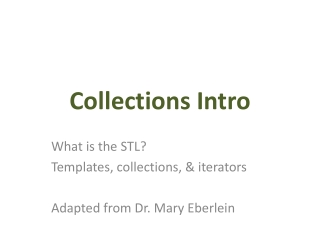DownloadDownload PresentationCollections Intro

# Collections Intro

Download Presentation## Collections Intro

- - - - - - - - - - - - - - - - - - - - - - - - - - - E N D - - - - - - - - - - - - - - - - - - - - - - - - - - -
##### Presentation Transcript

1. Collections Intro What is the STL? Templates, collections, & iterators Adapted from Dr. Mary Eberlein

2. Data Structures • Collection or data structure: an object that stores data • elements: objects that are stored • collections can be ordered or unordered • some collections allow duplicate elements • Common operations: insert, size, isEmpty... • Good to know how to build collections (implementation) • Often use an available collection rather than implementing it yourself (and learn to use it effectively)

3. Type Parameters (templates) Collection<type> name; • specify type of elements in triangle brackets • Called a type parameter – you get a parameterized class, or template class • type can be any data type: int, char, string, user-defined type... • Example: vector<int> v;

4. STL: Standard Template Library • STL: library of collections and algorithms for C++ • Many use templates • container classes (set, list, stack, vector, queue...) • algorithms • iterators

5. VECTOR

6. Won't Arrays Do? • Arrays: • fixed size – not easily resized • array doesn't "know" its own size • array index out of bounds errors not caught • don't support useful operations like: • inserting/deleting elements at front/middle/back of array • reversing order of elements • searching or sorting • preventing duplicate elements from being added

7. Vector • vector (aka list): collection of elements with 0-based indexing • add elements anywhere in vector • automatic resizing as needed • vector has a size (current # of elements) • template class – create vectors of different types • No need to allocate/delete memory for vector • Header: #include<vector> • Recall: all of standard library in namespace std • vector<int> myVector; // vector of ints • vector<string> strVec; // vector of strings

8. Example C++ Vector Example: #include <vector> using namespace std; ... int size = 10; // 10 ints in array, init to 0 vector<int> myArr(size); for(inti = 0; i < size; i++) myArr[i] = i; // Don't need to delete! C++ Array Example: int size = 10; intmyArr; int *arrPtr = new int[size]; for(inti = 0; i < 10; i++){ myArr[i] = i; arrPtr[i] = i; } delete [] arrPtr; • Vector member function at(): • similar to indexing with [], but checks index validity • Resizing is handled for you when necessary

9. STL vector vector<int> v; // empty vector • Member functions: • push_back(value): insert element at the end of the vector • v.push_back(3); // 3 added at end of v • v.push_back(5); // 5 added at end of v • at(index): return element at specified index • int x = v.at(0); // 3 • size(): returns number of elements in vector • int numElts = v.size(); // 2 • front(): returns reference to first element of vector • int& num = v.front(); // 3 • back(): returns reference to last element of vector

10. STL iterator • iterator: object that iterates over a data structure • pointer-like object that can be incremented (++), dereferenced (*), compared to another iterator (!=) • stores current position within data structure • used to traverse over the elements in a collection • iterator generated by STL member functions like begin() and end() • begin():returns iterator pointing to 1st element of ordered collection (e.g., vector) • end():returns iterator which is past the end of the collection

11. Iterator • A collection has a begin and end iterator to front and back • Advance one element: iter++ • Go back one element: iter-- • Access element the iterator is next to: *iter vector<int> v; ... // loop over vector elements and print them for(vector<int>::iterator it = v.begin(); it != v.end(); it++) { cout << *it << endl; }

12. Iterator Example • // for-each loop – implicit iterator (C++11) vector<int> v; ... for(int k : v) { cout << k << endl; }

13. Iterator Example • Some container member functions take an iterator argument to indicate position • Example for vector: insert, erase vector<int> v; ... for(vector<int>::iterator it = v.end(); it != v.begin(); it--) { if(*it % 2 == 0) { it = v.erase(it); // delete element at this position } }

14. STL Algorithms • Many algorithms • Example: sorting vectors #include<algorithm> vector<string> vec; vec.push_back("hello"); vec.push_back("world"); vec.push_back("bye"); sort(v.begin(), v.end()); http://www.cplusplus.com/reference/algorithm/